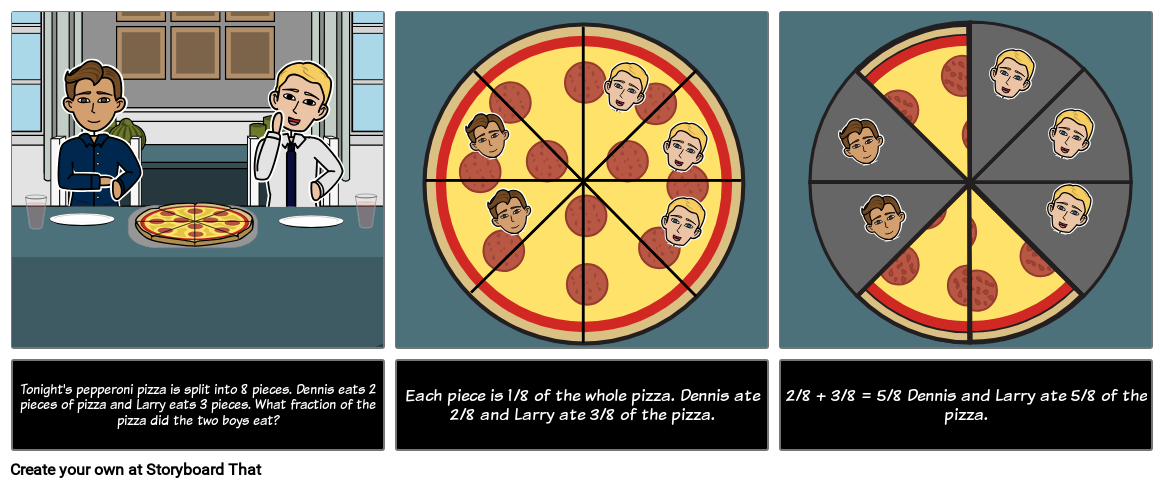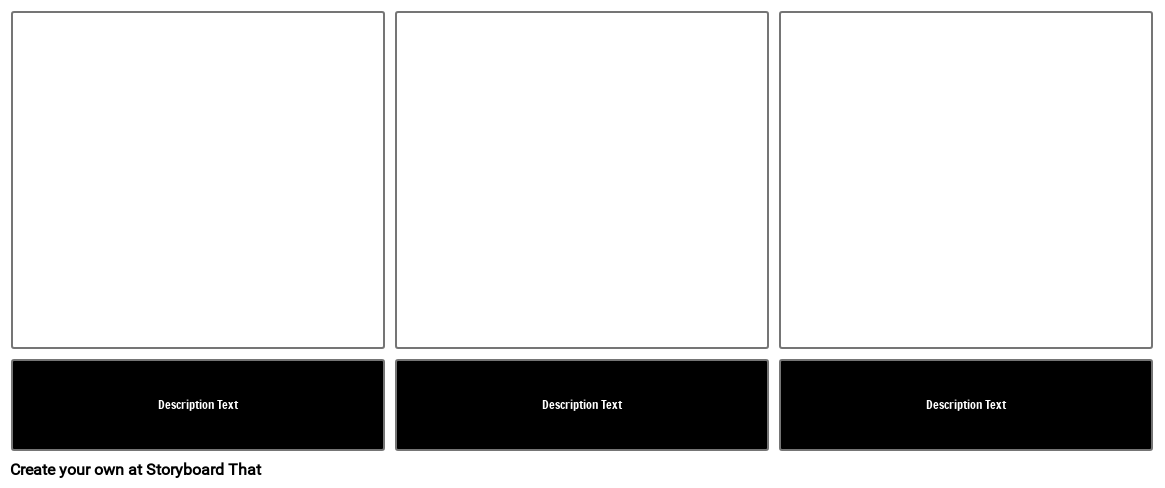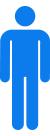Fractions - Eating a Pizza## Activity Overview

Before starting this activity, revisit what “numerator” and “denominator” mean, stressing that the denominator gives the name to the fraction. When adding fractions, you are adding parts; as long as you are adding the same kind of parts (common denominator), you only need to worry about how many parts in all. In this activity, students will add or subtract two fractions using visuals to help explain the process.

Give students examples of when you might add parts together, but be careful of how you word your questions!

“Dennis ate 2 pieces of pizza and Larry ate 2 pieces of pizza. How much pizza did they eat?”

Is the answer four pieces? Four-eighths? All of the pizza?

Be explicit in the question or example. Identify how many pieces make up the whole. Use precise language when asking the question, such as “what fraction” or “how many pieces”, so students know what they are looking for.

By rewording the story, you can use the same example for subtraction. You can change the question to “what fraction of the pizza is left” or “how many pieces are left?”

Just as in subtraction with whole numbers, be sure students are writing the numbers in the correct order. Typically, students have not yet encountered improper fractions, and certainly not negative numbers. The larger fraction goes first in the number sentence: 3/4 - 1/4 = 2/4.

Lesson Plan Reference

Difficulty Level 3 (Developing to Mastery)

Type of Assignment Individual

Common Core Standards
• [Math/Content/3/NF/A/1] Understand a fraction 1/b as the quantity formed by 1 part when a whole is partitioned into b equal parts; understand a fraction a/b as the quantity formed by a parts of size 1/b.

## Template and Class Instructions

(These instructions are completely customizable. After clicking "Use This Assignment With My Students", update the instructions on the Edit Tab of the assignment.)

#### Student Instructions

Practice adding and subtracting fractions with common denominators based on the question in the first cell.

1. Click "Start Assignment".
2. Read the question in the first cell carefully.
3. In the second cell, create a visual representation of the question and identify the different fractions you will be using.
4. In the third cell, create a visual representation of the answer and show how you got the answer.
5. Save and submit your storyboard.
Blank Cell with Description## Education Pricing

This pricing structure is only available to academic institutions. Storyboard That accepts purchase orders.#### Single Teacher

As low as /month#### Department

As low as /month#### School/District

As low as /month

*(This will start a 2-Week Free Trial - No Credit Card Needed)
##### Over 15 Million Storyboards Created